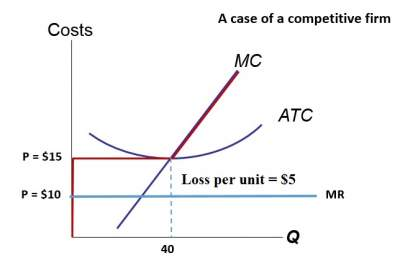# Exercise-2 on Identifying a firm's profit: Solutions

Question: As illustrated in the figure, determine this firm's total loss.Solution:
Total loss = (ATC − P) × Q = \$5 × 40 = \$200